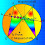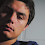## Sunday, May 18, 2008

### Elearn Geometry Problem 7See complete Problem 7 at:
www.gogeometry.com/problem/problem007.htm

Triangle, Cevian, Angles, Congruence. Level: High School, SAT Prep, College geometry

1.trazar la ceviana exterior BE (que corta a la prolongacion de AC en E)
Si el angulo EBC=x
entonces el angulo BEC=3x...(1)
Ademas los triangulos ABC y BED son congruentes
y de (1) se tiene que: el angulo ABC=3x
Finalmente en el triangulo ABC
3x+4x+3x=180º
10x=180º
x=18º

atte:tino

2.http://geometri-problemleri.blogspot.com/2009/10/problem-34-ve-cozumu.html

3.http://ahmetelmas.files.wordpress.com/2010/05/cozumlu-ornekler.pdf

4.Given:
ABC=A+B+C
ABC=180 A=3x B=x+2x C=4x
Solution:
ABC=A+B+C
180=3x+x+2x+4x
180=10x
180/10=10x/10
18=x

A=3x B=x+2x C=4x
A=3(18) B=18+2(18) C=4(18)
A=54 B=18+36 C=72
B=54

180=54+54+72
180=180

5.To Jonathan (Problem 7): Why B=x+2x?

6.Video-solution to the problem by me:

Greetings.

7.1.Triangles in the first step can not be congruent by AAS
there is no criteria of AAS for congruence

2.There is no rule of AAS congruence, side common between angles must be same...................

8.Sea E el punto en la prolongación de A-C tal que CE = AD
Sea F tal que F-E es paralela a D-B y además EF=DB
Con esto se arma un rombo DBFE
La diagonal B-E corta a C-F en un punto G
Esta diagonal también es bisectriz por lo que ang(GEC)=3α, lo que hace que
△CGE y △BGF sean isósceles.
Como BFEC es un paralelogramo recto se deduce que:
ang(ECB) = ang(FEC)
Pero esto implica:
6α=180°-4α
α=18° Q.E.D.

9.Construct isoceles Tr. ABE where E is on AC. Then Tr. BDE is isoceles and so DE = BD= AC

Hence Tr.s ABC & BED are congruent SAS from which it follows that Tr. BDC is isoceles with internal angles adding upto 10x and so x=18

Sumith Peiris
Moratuwa
Sri Lanka

10.Construct point E such that BA=BE (hence triangle ABE is formed and it is an isosceles triangle, where <BAE=<BEA=3x). Additionally, E lies on AC produced.
Consider triangle ABC and EBD.
<ABD=180-9x
<ABE=180-6x
Hence <EBC=(180-6x)-2x-(180-9x)
<EBC=x
Hence <DBE=3x
Since <DBE=<DEB=3x, DB=DE
Since AC=DB (given), AC=DE

1.BA=BE (from above)
2.<BAC=<BED=3x (proven)
3.AC=ED (proven)
Hence triangle ABC is congruent to EBD (SAS)
Hence, BD=BC
<BDC=4x
In triangle BCD,
2x+4x+4x=180
x=18

11.By the law of sines,
sin(∠BDC):sin(∠C) = BC:BD = BC:AC = sin(∠A):sin(∠ABC).
That is,
sin(180°-6x):sin(4x) = sin(3x):sin(180°-7x),
thus,
sin(6x):sin(4x) = sin(3x):sin(7x).
This implies
sin(7x)sin(6x) = sin(4x)sin(3x)
By the product-to-sum identities,
-(1/2)(cos(13x) - cos(x)) = -(1/2)(cos(7x) - cos(x)),
which can be reduced to
cos(13x) - cos(7x) = 0 (since cos(x) = 0 is not valid).
Then by the sum-to-product identity,
-2sin(10x)sin(3x) = 0
which suggests
sin(10x) = 0 or sin(3x) = 0.
This means x is either a multiple of 18° or a multiple of 60°.
Since ∠A = 3x, ∠C = 4x, and ∠ABC = 180°-7x must all be in (0°, 180°), we must have x = 18°.

12.13.Construct H projection of B on AC
Construct E on AC such as DE=AC=BD
Then C and D are symmetrical with respect to H, thus <BDC=<BCD=4x, finally, in isoceles triangle ∆DBC:
180°=4x+4x+2x=10x and x=18°

14.Grab a pen and a piece of paper, and illustrate along.

Firstly, add point E to the figure and connect it to points B and C such that points A, C and E are collinear and angle BEC = 3x.

We can calculate that:
> Angle BCE = 180° - 4x
> Angle CBE = 180° - (180° - 4x) - 3x = x
> Angle DBE = 2x + x = 3x = angle BEC
> Triangle BDE is isosceles because angle BEC = angle DBE
> Line DE = line BD since triangle BDE is isosceles.
> Line DE = line BD = line AC
> Triangle ABE is isosceles since angles BAD and BEC are equal.
> Line AB = line BE since triangle ABE is isosceles.

Now we solve the problem:
> Line AE - line AC = line AE - line DE
> Line CE = line AD
> Triangles ABD and BCE are congruent by SAS:
· Line AB = line BE
· Angle BAD = angle BEC
· Line AD = line CE
> Angle ABD = 180° - 2x - 3x - 4x = 180° - 9x
> Since triangles ABD and BCE are congruent, angles ABD and CBE are equal.
> 180° - 9x = x
> 10x = 180°

Therefore x = 180°/10 = 18°

15.Construct point E such that AB=BE
Then,<CBE=x and <DBE=2x+x=3x

In ΔΒCE,
<DBE=<DEB=3x
So,BD=DE

Since AC=BD
We can say that AC=BD=DE

Now,
AE-DE=AE-AC

In ΔABD and ΔBCE,
AB=BE

We get these two triangles congruent.
So,<CBE=x=<ABD

Hence,<ABC=x+2x=3x

In triangle ABC,
3x+3x+4x=180
x=18 and we are done

Partho Saha